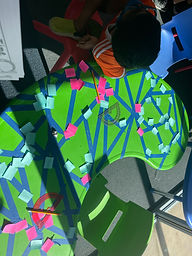## Mr. Haidi

### Target 1​

###### Lesson Type:

New

Geometry

:

Shape

Measure, draw, and classify angles.

###### 1:

Accurately measure angles.

###### 2:

Identify angles as acute, obtuse, or right.

###### 3:

Identify angle types and estimate their measurement.

###### 4:

Understand that an angle is the union of two rays with a common endpoint (vertex).

###### 5:

Understand that angles are measured in degrees (from 0 to 360).

4th

###### Vocabulary:

Obtuse, Acute, Right angles.

Activities:

Students were given a table filled with numerous intersecting rays and were asked to measure and identify the kind of angles present.### Home Exploration

###### Guiding Questions:## Absent Students:

### Target 2

:

###### 1:

Understand that time is the duration of an event from beginning to end.

###### 2:

Understand that elapsed time can be found by find the total amount of time that passes between the starting and ending time of an event.

###### 3:

Solve addition and subtraction problems with time duration.

4th

###### Vocabulary:

Activities:

Students solved various world problems involving elapsed time.### Home Exploration

###### Guiding Questions:### Target 3

:

###### Vocabulary:

Activities:### Home Exploration